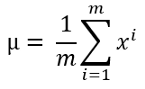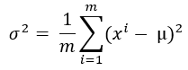# A Complete Anomaly Detection Algorithm From Scratch in Python: Step by Step GuideAnomaly Detection Algorithm Using the Probabilities. In this article, I will explain the process of developing an anomaly detection algorithm from scratch in Python.

Anomaly detection can be treated as a statistical task as an outlier analysis. But if we develop a machine learning model, it can be automated and as usual, can save a lot of time. There are so many use cases of anomaly detection. Credit card fraud detection, detection of faulty machines, or hardware systems detection based on their anomalous features, disease detection based on medical records are some good examples. There are many more use cases. And the use of anomaly detection will only grow.

In this article, I will explain the process of developing an anomaly detection algorithm from scratch in Python.

### The Formulas and Process

This will be much simpler compared to other machine learning algorithms I explained before. This algorithm will use the mean and variance to calculate the probability for each training data.

If the probability is high for a training example, it is normal. If the probability is low for a certain training example it is an anomalous example. The definition of high and low probability will be different for the different training sets. We will talk about how to determine that later.

If I have to explain the working process of anomaly detection, that’s very simple.

1. Calculate the mean using this formula:Here m is the length of the dataset or the number of training data and xi is a single training example. If you have several training features, most of the time you will have, the mean needs to be calculated for each feature.

2. Calculate the variance using this formula:Here, mu is the calculated mean from the previous step.

## Most popular Data Science and Machine Learning courses — July 2020

Most popular Data Science and Machine Learning courses — August 2020. This list was last updated in August 2020 — and will be updated regularly so as to keep it relevant

## Artificial Intelligence vs Machine Learning vs Data Science

Artificial Intelligence, Machine Learning, and Data Science are amongst a few terms that have become extremely popular amongst professionals in almost all the fields.

## Pipelines in Machine Learning | Data Science | Machine Learning | Python

Machine Learning Pipelines performs a complete workflow with an ordered sequence of the process involved in a Machine Learning task. The Pipelines can also

## Data Science Projects | Data Science | Machine Learning | Python

Practice your skills in Data Science with Python, by learning and then trying all these hands-on, interactive projects, that I have posted for you.

## Data Science Projects | Data Science | Machine Learning | Python

Practice your skills in Data Science with Python, by learning and then trying all these hands-on, interactive projects, that I have posted for you.